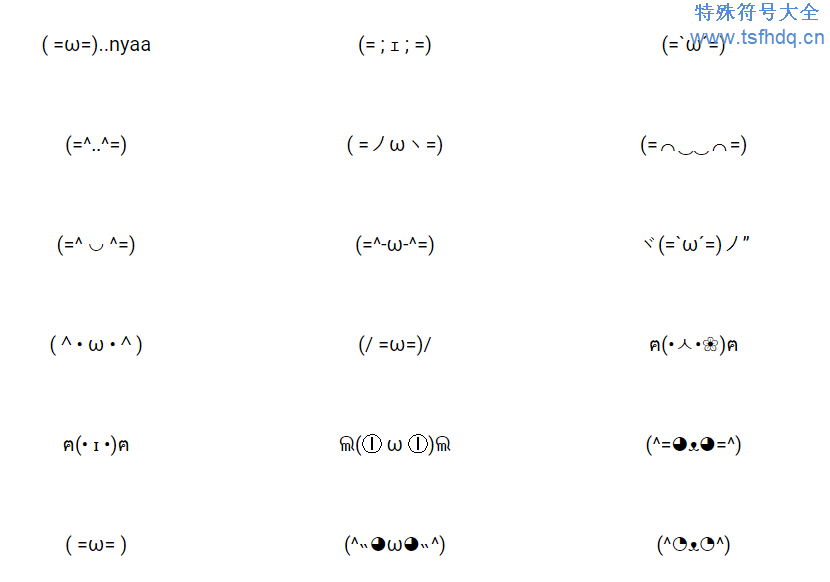# 可爱符号ヽ( ･∀･)ﾉ_θ彡☆Σ(ノ `Д´)ノ

(*´∇`)┌θ☆(ﾉ>_<)ﾉ

( ￣ω￣)ノﾞ⌒☆ﾐ(o _ _)o

(*`0´)θ☆(メ°皿°)ﾉ

(o¬‿¬o )...☆ﾐ(*x_x)

(╬￣皿￣)=○#(￣#)3￣)

(; -_-)――――――C<―_-)

<( ￣︿￣)︵θ︵θ︵☆(>口<-)

(￣ε(#￣)☆╰╮o(￣▽￣///)

ヽ(>_<ヽ) ―⊂|=0ヘ(^‿^ )

ヘ(>_<ヘ) ￢o(￣‿￣ﾒ)

,,((( ￣□)_/ \_(○￣ ))),,

(҂` ﾛ ´)︻デ═一 \(º □ º l|l)/

(╯°Д°)╯︵ /(.□ . \)

(¬_¬'')ԅ(￣ε￣ԅ)

/( .□.)\ ︵╰(°益°)╯︵ /(.□. /)

(ﾉ-.-)ﾉ….((((((((((((●~* ( >_<)

!!(ﾒ￣ ￣)_θ☆°0°)/

(`⌒*)O-(`⌒´Q)

(((ง’ω’)و三 ง’ω’)ڡ≡ ☆⌒ﾐ((x_x)

(งಠ_ಠ)ง σ( •̀ ω •́ σ)

(っ•﹏•)っ ✴==≡눈٩(`皿´҂)ง

(｢• ω •)｢ (⌒ω⌒`)

( °ᴗ°)~ð (/❛o❛)